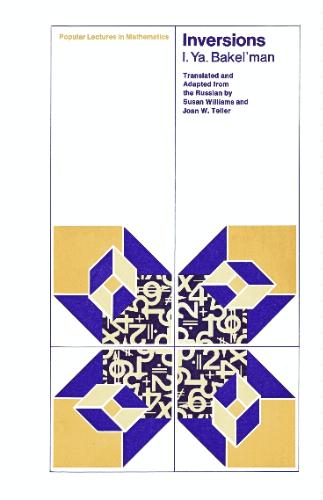# [share_ebook] Inversions

#### Tag: Mathematics

Posted on 2008-03-22, by MathTutor.

Description• Author: I. Ya. Bakelman
• Publisher: University Of Chicago Press
• Publish Date: 1975
• ISBN: 0226034992
• Pages: 77

Popular Lectures in Mathematics

In this book, I. Ya. Bakel'man introduces inversion transformations in the Euclidean plane and discusses the interrelationships among more general mathematical concepts. The author begins by defining and giving examples of the concept of a transformation in the Euclidean plane, and then explains the "point of infinity" and the "stereographic projection" of the sphere onto the plane. With this preparation, the student is capable of applying the theory of inversions to classical construction problems in the plane.

The author also discusses the theory of pencils of circles, and he uses the acquired techniques in a proof of Ptolemy's theorem. In the final chapter, the idea of a group is introduced with aplications of group theory to geometry. The author demonstrates the group-theoretic basis for the distinction between Euclidean and Lobachevskian geometry.

English, Russian (translation)

7619 dl's @ 2365 KB/s
5649 dl's @ 2387 KB/s
5886 dl's @ 3170 KB/s

Search More...
[share_ebook] InversionsRelated Books